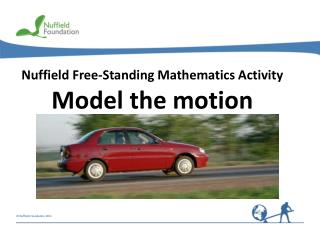DownloadDownload PresentationNuffield Free-Standing Mathematics Activity

Nuffield Free-Standing Mathematics Activity

Download PresentationNuffield Free-Standing Mathematics Activity

- - - - - - - - - - - - - - - - - - - - - - - - - - - E N D - - - - - - - - - - - - - - - - - - - - - - - - - - -
Presentation Transcript

1. Nuffield Free-Standing Mathematics Activity Model the motion

2. Model the motion How can we model motion? What would a velocity–time graph look like for each of these? What would a displacement–time graph look like? Are there any connections between the graphs?

3. v (ms-1) x (m) 180 9 x x 150 t t 6 120 0 10 20 30 t (s) 90 60 3 30 0 10 20 30 t(s) Sprinter runs at 9 ms-1 for 10 seconds, stops for 10 seconds, then runs at 9 ms-1 for another 10 seconds in the same direction. Think about: What would a velocity–time graph look like? Think about: What would a displacement–time graph look like? = 9 Gradient = = 10  9 = 90 Area Area under the velocity–time graph gives the displacement The gradient of the displacement–time graph gives the velocity

4. x(m) 90 60 x x 30 0 t t 10 20 30 t(s) v(ms-1) 9 6 3 0 10 20 30 t(s) – 3 – 6 – 9 Sprinter runs at 9 ms-1 for 10 seconds, stops for 10 seconds, then takes 10 seconds to run back to his starting point. Think about: What would a velocity-time graph and a displacement-time graph look like? Area under the velocity–time graph gives the displacement The gradient of the displacement–time graph gives the velocity Gradient = 9 ms-1 Area = 90 m Total = 0 Gradient Area = –90 m = –9 ms-1

5. v(ms-1) x (m) 200 20 B A A B t (s) 10 100 Area A = 160 m Gradient A Area B Gradient B varies from 20 to 0 ms-1 0 0 12 12 2 4 6 10 2 4 6 10 8 8 t(s) A mechanic drives a car at a steady speed of 20 ms-1 for 8 seconds then puts on the brakes to come to a halt 4 seconds later. Area under the velocity–time graph gives the displacement The gradient of the displacement–time graph gives the velocity Think about: What would a velocity–time graph and a displacement–time graph look like this time? = 20 ms-1 = 40 m

6. x x t t Gradient Gradient x (m) v (ms-1) 1200 10 1000 5 800 t(min) 600 0 12 2 4 6 10 8 400 – 5 200 0 – 10 2 4 6 8 10 12 t (min) A driver drives her car 1.2 km to a garage, spends 8 minutes checking her tyres then drives the car back home again. Think about: Can you sketch a displacement-time graph? Gradient gives the velocity Area gives the displacement Think about: Can you sketch a velocity-time graph? = 1200 m Area = 10  120 = 10 ms-1 = – 1200 m Area = – 10  120 = – 10 ms-1

7. x (m) v (ms-1) 60 10 B 50 A A B 40 5 30 20 Gradient A varies from 0 to 10 ms-1 Area A Gradient B varies from 10 to 0 ms-1 Area B 10 0 0 12 12 2 2 4 4 6 6 10 10 8 8 t (s) t(s) The distance between two bus stops is 60 metres. A bus sets off from the first bus stop and reaches a speed of 10 ms-1 before braking to stop at the second bus stop. Think about: Can you sketch a velocity-time graph? Think about: What is the displacement in each part? Area gives the displacement Gradient gives the velocity Think about: What does the displacement-time graph look like? = 40 m = 20 m

8. v (ms-1) 2 1 x 0 10 15 t(s) 20 5 t – 1 x – 2 t Gradient Gradient x (m) 20 10 0 10 15 20 5 t (s) – 10 A remote controlled car reverses 10 metres then travels 30 metres forward at the same speed. This takes a total of 20 seconds. Think about: What would a displacement-time graph and a velocity-time graph look like this time? Gradient gives the velocity Area gives the displacement Area = – 2  5 = –10 m = –2 ms-1 Area = 2  15 = 30 m Total = 20 m = 2 ms-1

9. Model the motion Reflect on your work What is the difference between distance and displacement? Is speed the same as velocity? How are velocity–time graphs and displacement–time graphs related? How realistic are the graphs as models of the motion described? What would more realistic graphs look like?